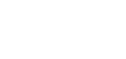## ReleasesPowerTools for Salesforce

Salesforce users can now leverage the advanced capabilities of Apptigent PowerTools in their sales automation solutions. Available in all Salesforce versions directly from AppExchange, PowerTools adds easily configurable drag-and-drop actions to Visual Flow and Process Builder, complete with custom Apex objects for pre-populated picklist controls in screens and forms.

General Availability: 01.01.2021PowerTools PRO 1.3.0

PowerTools PRO for Microsoft Power Platform receives a major update this quarter, with over twenty essential new actions, including speech to text, QR code generation, string to file, stock market quotes, and more!  See the feature table below for complete details.

General Availability: 01.12.2021PowerTools for Nintex 1.1.0

PowerTools for Nintex receives a major update this quarter, with over a twenty essential new actions, including speech to text, QR code generation, string to file, stock market quotes, and more! See the feature table below for complete details.

General Availability: 01.01.2021

 Finance Stock PricesGet current and historical stock price Market IndexGet current and historical market index information Math Calculate AdditionCalculate the sum of two numbers Calculate SubtractionCalculate the difference between two numbers Calculate MultiplicationCalculate the product of two numbers Calculate DivisionCalculate the quotient of two numbers Calculate ModuloCalculate the remainder of dividing two numbers Calculate Nth RootCalculate the n-th root of a number Calculate Square RootCalculate the square root of a number Calculate LogarithmCalculate the logarithm of a number Calculate SineCalculate the sine value of an angle Calculate CosineCalculate the cosine value of an angle Text Generate HashGenerate a hash value from a string Verify HashVerify a hashed value against the original source string Speech to TextConvert audio file to text String to FileConvert text string to file Files Rotate ImageRotate an image by specified number of degrees Flip ImageFlip an image (horizontal or vertical) Watermark ImageAdd watermark text to an image Crop ImageCrop an image Generate QR CodeGenerate a QR code image File to StringConvert a file to a Base64 string Data Query JSONQuery a JSON object using a JSONPath expression Query XMLQuery an XML string using an XPath expressionPowerTools Developer 1.1.0

PowerTools Developer Edition receives a major update this quarter, with over twenty essential new actions, including speech to text, QR code generation, string to file, stock market quotes, and more! See the feature table below for complete details.

General Availability: 01.01.2021

 Finance Stock PricesGet current and historical stock price Market IndexGet current and historical market index information Math Calculate AdditionCalculate the sum of two numbers Calculate SubtractionCalculate the difference between two numbers Calculate MultiplicationCalculate the product of two numbers Calculate DivisionCalculate the quotient of two numbers Calculate ModuloCalculate the remainder of dividing two numbers Calculate Nth RootCalculate the n-th root of a number Calculate Square RootCalculate the square root of a number Calculate LogarithmCalculate the logarithm of a number Calculate SineCalculate the sine value of an angle Calculate CosineCalculate the cosine value of an angle Text Generate HashGenerate a hash value from a string Verify HashVerify a hashed value against the original source string Speech to TextConvert audio file to text String to FileConvert text string to file Files Rotate ImageRotate an image by specified number of degrees Flip ImageFlip an image (horizontal or vertical) Watermark ImageAdd watermark text to an image Crop ImageCrop an image Generate QR CodeGenerate a QR code image File to StringConvert a file to a Base64 string Data Query JSONQuery a JSON object using a JSONPath expression Query XMLQuery an XML string using an XPath expression